xFundamental theorem of arithmeticEncyclopedia
In number theory
Number theory
Number theory is a branch of pure mathematics devoted primarily to the study of the integers. Number theorists study prime numbers as well...

, the fundamental theorem of arithmetic (or the unique-prime-factorization theorem) states that any integer
Integer
The integers are formed by the natural numbers together with the negatives of the non-zero natural numbers .They are known as Positive and Negative Integers respectively...

greater than 1 can be written as a unique product (up to
Up to
In mathematics, the phrase "up to x" means "disregarding a possible difference in  x".For instance, when calculating an indefinite integral, one could say that the solution is f "up to addition by a constant," meaning it differs from f, if at all, only by some constant.It indicates that...

ordering of the factor
Factorization
In mathematics, factorization or factoring is the decomposition of an object into a product of other objects, or factors, which when multiplied together give the original...

s) of prime number
Prime number
A prime number is a natural number greater than 1 that has no positive divisors other than 1 and itself. A natural number greater than 1 that is not a prime number is called a composite number. For example 5 is prime, as only 1 and 5 divide it, whereas 6 is composite, since it has the divisors 2...

s. For example,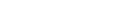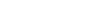are two numbers satisfying the hypothesis
Hypothesis
A hypothesis is a proposed explanation for a phenomenon. The term derives from the Greek, ὑποτιθέναι – hypotithenai meaning "to put under" or "to suppose". For a hypothesis to be put forward as a scientific hypothesis, the scientific method requires that one can test it...

of the theorem
Theorem
In mathematics, a theorem is a statement that has been proven on the basis of previously established statements, such as other theorems, and previously accepted statements, such as axioms...

that can be written as the product of prime numbers.

Proof of existence
Constructive proof
In mathematics, a constructive proof is a method of proof that demonstrates the existence of a mathematical object with certain properties by creating or providing a method for creating such an object...

of a prime factorization is straightforward; proof of uniqueness is more challenging. Some proof
Mathematical proof
In mathematics, a proof is a convincing demonstration that some mathematical statement is necessarily true. Proofs are obtained from deductive reasoning, rather than from inductive or empirical arguments. That is, a proof must demonstrate that a statement is true in all cases, without a single...

s use the fact that if a prime number p divides
Divisor
In mathematics, a divisor of an integer n, also called a factor of n, is an integer which divides n without leaving a remainder.-Explanation:...

the product of two natural number
Natural number
In mathematics, the natural numbers are the ordinary whole numbers used for counting and ordering . These purposes are related to the linguistic notions of cardinal and ordinal numbers, respectively...

s a and b, then p divides either a or b, a statement known as Euclid's lemma
Euclid's lemma
In mathematics, Euclid's lemma is an important lemma regarding divisibility and prime numbers. In its simplest form, the lemma states that a prime number that divides a product of two integers must divide one of the two integers...

. Since multiplication on the integers is both commutative
Commutativity
In mathematics an operation is commutative if changing the order of the operands does not change the end result. It is a fundamental property of many binary operations, and many mathematical proofs depend on it...

and associative
Associativity
In mathematics, associativity is a property of some binary operations. It means that, within an expression containing two or more occurrences in a row of the same associative operator, the order in which the operations are performed does not matter as long as the sequence of the operands is not...

, it does not matter in what way we write a number greater than 1 as the product of primes; it is common to write the (prime) factors in the order of smallest to largest.

Some natural extensions of the hypothesis of this theorem allow any non-zero integer to be expressed as the product of "prime numbers" and "invertibles". For example, 1 and −1 are allowed to be factors of such representations (although they are not considered to be prime). In this way, one can extend the Fundamental Theorem of Arithmetic to any Euclidean domain
Euclidean domain
In mathematics, more specifically in abstract algebra and ring theory, a Euclidean domain is a ring that can be endowed with a certain structure – namely a Euclidean function, to be described in detail below – which allows a suitable generalization of the Euclidean algorithm...

or principal ideal domain
Principal ideal domain
In abstract algebra, a principal ideal domain, or PID, is an integral domain in which every ideal is principal, i.e., can be generated by a single element. More generally, a principal ideal ring is a nonzero commutative ring whose ideals are principal, although some authors refer to PIDs as...

bearing in mind certain alterations to the hypothesis of the theorem. A ring
Ring (mathematics)
In mathematics, a ring is an algebraic structure consisting of a set together with two binary operations usually called addition and multiplication, where the set is an abelian group under addition and a semigroup under multiplication such that multiplication distributes over addition...

in which the Fundamental Theorem of Arithmetic holds is called a unique factorization domain
Unique factorization domain
In mathematics, a unique factorization domain is, roughly speaking, a commutative ring in which every element, with special exceptions, can be uniquely written as a product of prime elements , analogous to the fundamental theorem of arithmetic for the integers...

.

Many authors assume 1 to be a natural number that has no prime factorization. Thus Theorem 1 of takes the form, "Every positive integer, except 1, is a product of one or more primes," and Theorem 2 (their "Fundamental") asserts uniqueness. By convention, the number 1 is not itself prime, but since it is the product of no numbers, it is often convenient to include it in the theorem by the empty product
Empty product
In mathematics, an empty product, or nullary product, is the result of multiplying no factors. It is equal to the multiplicative identity 1, given that it exists for the multiplication operation in question, just as the empty sum—the result of adding no numbers—is zero, or the additive...

rule. (See, for example, Calculating the gcd.)

## Applications

The fundamental theorem of arithmetic establishes the importance of prime numbers. Prime numbers are the basic building blocks of any positive integer, in the sense that each positive integer can be constructed from the product of primes with one unique construction. Finding the prime factorization of an integer allows derivation of all its divisors, both prime and non-prime. This property of uniqueness is powerful, as the factorization can be thought of as a key for a number. This is why it can be used to build powerful encryption techniques such as RSA.

For example, the above factorization of 6936 shows that any positive divisor of 6936 must have the form 2a × 3b × 17c, where a takes one of the 4 values in {0, 1, 2, 3}, where b takes one of the 2 values in {0, 1}, and where c takes one of the 3 values in {0, 1, 2}. Multiplying the numbers of independent options together produces a total of 4 × 2 × 3 = 24 positive divisors.

Once the prime factorizations of two numbers are known, their greatest common divisor
Greatest common divisor
In mathematics, the greatest common divisor , also known as the greatest common factor , or highest common factor , of two or more non-zero integers, is the largest positive integer that divides the numbers without a remainder.For example, the GCD of 8 and 12 is 4.This notion can be extended to...

and least common multiple
Least common multiple
In arithmetic and number theory, the least common multiple of two integers a and b, usually denoted by LCM, is the smallest positive integer that is a multiple of both a and b...

can be found quickly. For instance, from the above it is shown that the greatest common divisor of 6936 and 1200 is 23 × 3 = 24. However, if the prime factorizations are not known, the use of the Euclidean algorithm
Euclidean algorithm
In mathematics, the Euclidean algorithm is an efficient method for computing the greatest common divisor of two integers, also known as the greatest common factor or highest common factor...

generally requires much less calculation than factoring the two numbers.

The fundamental theorem ensures that additive
In mathematics the term additive function has two different definitions, depending on the specific field of application.In algebra an additive function is a function that preserves the addition operation:for any two elements x and y in the domain. For example, any linear map is additive...

and multiplicative
Multiplicative function
In number theory, a multiplicative function is an arithmetic function f of the positive integer n with the property that f = 1 and whenevera and b are coprime, then...

arithmetic function
Arithmetic function
In number theory, an arithmetic function is a real or complex valued function ƒ defined on the set of natural numbers In number theory, an arithmetic (or arithmetical) function is a real or complex valued function ƒ(n) defined on the set of natural numbers In number theory, an arithmetic (or...

s are completely determined by their values on the powers of prime numbers.

It may be important to note that Egyptians like Ahmes
Ahmes
Ahmes was an ancient Egyptian scribe who lived during the Second Intermediate Period and the beginning of the Eighteenth Dynasty . He wrote the Rhind Mathematical Papyrus, a work of Ancient Egyptian mathematics that dates to approximately 1650 BC; he is the earliest contributor to mathematics...

used earlier practical aspects of the factoring, such as the least common multiple
Least common multiple
In arithmetic and number theory, the least common multiple of two integers a and b, usually denoted by LCM, is the smallest positive integer that is a multiple of both a and b...

, allowing a long tradition to develop, as formalized by Euclid, and rigorously proven by Carl Friedrich Gauss
Carl Friedrich Gauss
Johann Carl Friedrich Gauss was a German mathematician and scientist who contributed significantly to many fields, including number theory, statistics, analysis, differential geometry, geodesy, geophysics, electrostatics, astronomy and optics.Sometimes referred to as the Princeps mathematicorum...

.

Although at first sight the theorem seems 'obvious', it does not hold in more general number systems, including many rings of algebraic integers. This was first pointed out by Ernst Kummer
Ernst Kummer
Ernst Eduard Kummer was a German mathematician. Skilled in applied mathematics, Kummer trained German army officers in ballistics; afterwards, he taught for 10 years in a gymnasium, the German equivalent of high school, where he inspired the mathematical career of Leopold Kronecker.-Life:Kummer...

in 1843, in his work on Fermat's Last Theorem
Fermat's Last Theorem
In number theory, Fermat's Last Theorem states that no three positive integers a, b, and c can satisfy the equation an + bn = cn for any integer value of n greater than two....

. The recognition of this failure is one of the earliest developments in algebraic number theory
Algebraic number theory
Algebraic number theory is a major branch of number theory which studies algebraic structures related to algebraic integers. This is generally accomplished by considering a ring of algebraic integers O in an algebraic number field K/Q, and studying their algebraic properties such as factorization,...

.

## Euclid's proof

The proof consists of two steps. In the first step every number is shown to be a product of zero or more primes. In the second step, the proof shows that any two representations may be unified into a single representation.

### Non-prime composite numbers

Proof by contradiction via minimal counterexample
Minimal counterexample
In mathematics, the method of considering a minimal counterexample combines the ideas of inductive proof and proof by contradiction. Abstractly, in trying to prove a proposition P, one assumes that it is false, and that therefore there is at least one counterexample...

: Suppose there were positive integers which cannot be written as a product of primes. Then by the well-ordering principle
Well-ordering principle
The "well-ordering principle" is a property of ordered sets. A set X is well ordered if every subset of X has a least element. An example of a well ordered set is the set of natural numbers. An example of set that is not well ordered is the set of integers...

there must be a smallest such number, n. Because of the empty-product convention above, n cannot be 1. Also, n cannot be a prime number, since any prime number is a product of a single prime: itself. So n must be a composite number. Thus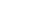where both a and b are positive integers smaller than n. Since n is the smallest number which cannot be written as a product of primes, both a and b can be written as products of primes. But thencan be written as a product of primes as well, simply by combining the factorizations of a and b; but this contradicts our supposition. Hence, all positive integers can be written as a product of primes.

### Proof of uniqueness

The key step in proving uniqueness is
Euclid's proposition 30 of book 7 (known as Euclid's lemma
Euclid's lemma
In mathematics, Euclid's lemma is an important lemma regarding divisibility and prime numbers. In its simplest form, the lemma states that a prime number that divides a product of two integers must divide one of the two integers...

), which states that, for any prime number p and any natural numbers a, b: if p divides ab then p divides a or p divides b.

This may be proved as follows:
• Suppose that a prime p divides ab (where a, b are natural numbers) but does not divide a. We must prove that p divides b.
• Since p does not divide a, and p is prime, the greatest common divisor
Greatest common divisor
In mathematics, the greatest common divisor , also known as the greatest common factor , or highest common factor , of two or more non-zero integers, is the largest positive integer that divides the numbers without a remainder.For example, the GCD of 8 and 12 is 4.This notion can be extended to...

of p and a is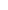.
• By Bézout's identity
Bézout's identity
In number theory, Bézout's identity for two integers a, b is an expressionwhere x and y are integers , such that d is a common divisor of a and b. Bézout's lemma states that such coefficients exist for every pair of nonzero integers...

, it follows that for some integers x, y (possibly negative),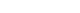• Multiplying both sides by b,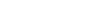• Since p divides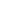, p divides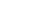• Since p divides both summands on the left, p divides b.

A proof of the uniqueness of the prime factorization of a given integer proceeds as follows.
Let s be the smallest natural number that can be written as (at least) two different products
Product (mathematics)
In mathematics, a product is the result of multiplying, or an expression that identifies factors to be multiplied. The order in which real or complex numbers are multiplied has no bearing on the product; this is known as the commutative law of multiplication...

of prime numbers. Denote these two factorizations of s as p1···pm and q 1···qn, such that s = p1p2···pm = q 1q2···qn.
Both q1 and q 2···qn must have unique prime factorizations (since both are smaller than s). By Euclid's proposition either p1 divides q1, or p1 divides q 2···qn. Therefore p1  =  qj (for some j). But by removing p1 and qj from the initial equivalence we have a smaller integer factorizable in two ways, contradicting our initial assumption. Therefore there can be no such s, and all natural numbers have a unique prime factorization.

### Alternate proof of uniqueness

Assume that s is the least integer that can be written as (at least) two different products
Product (mathematics)
In mathematics, a product is the result of multiplying, or an expression that identifies factors to be multiplied. The order in which real or complex numbers are multiplied has no bearing on the product; this is known as the commutative law of multiplication...

of prime numbers. Denote these two factorizations of s as p1···pm and q 1···qn, such that s = p1p2···pm = q1q2···qn.

No pi (with 1 ≤ i ≤ m) can be equal to any qj (with 1 ≤ j ≤ n), as there would otherwise be a smaller integer factorizable in two ways (by removing prime factors common in both products), violating the above assumption. Now it can be assumed without loss of generality
Without loss of generality
Without loss of generality is a frequently used expression in mathematics...

that p1 is a prime factor smaller than any q j (with 1 ≤ j ≤ n). Note that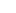divides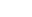. Sinceis smaller than s, it has an unique factorization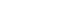. This is impossible.

## Canonical representation of a positive integer

Since the primes into which an integer can be factored need not be distinct, it follows from the Fundamental Theorem of Arithmetic that each integer n ≥ 2 can be represented as a product of prime powers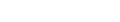that is,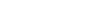where αi are positive integers and pi are primes satisfying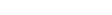.

This representation is called the canonical representation of n, or the standard form of n. Thus, 22·3, 24·34, and 22·5·11 are the canonical representations of 12, 1296, and 220 in that order. Each positive integer has exactly one such representation.

If, in a given problem, only one number is represented in this way, we usually require αi to be positive for each i, as above. However, for notational convenience when two or more numbers are involved, we sometimes allow some of the exponents to be zero. If α1 = 0, for example, the prime p1 simply does not occur in the canonical representation of n. This device makes it possible to write the canonical representation of any two positive integers so that they appear to involve the same prime factors even though they may, in fact, fail to have any nontrivial common factor. For example, we could write 12 = 22·3·50 and 20 = 22·30·5. And one could even write 1 = 20·30·50.

## Generalizations

The fundamental theorem of arithmetic generalizes to various contexts; for example in the context of ring theory
Ring theory
In abstract algebra, ring theory is the study of rings—algebraic structures in which addition and multiplication are defined and have similar properties to those familiar from the integers...

, where the field of algebraic number theory
Algebraic number theory
Algebraic number theory is a major branch of number theory which studies algebraic structures related to algebraic integers. This is generally accomplished by considering a ring of algebraic integers O in an algebraic number field K/Q, and studying their algebraic properties such as factorization,...

develops. A ring is said to be a unique factorization domain
Unique factorization domain
In mathematics, a unique factorization domain is, roughly speaking, a commutative ring in which every element, with special exceptions, can be uniquely written as a product of prime elements , analogous to the fundamental theorem of arithmetic for the integers...

if the Fundamental theorem of arithmetic (for non-zero elements) holds there. For example, any Euclidean domain
Euclidean domain
In mathematics, more specifically in abstract algebra and ring theory, a Euclidean domain is a ring that can be endowed with a certain structure – namely a Euclidean function, to be described in detail below – which allows a suitable generalization of the Euclidean algorithm...

or principal ideal domain
Principal ideal domain
In abstract algebra, a principal ideal domain, or PID, is an integral domain in which every ideal is principal, i.e., can be generated by a single element. More generally, a principal ideal ring is a nonzero commutative ring whose ideals are principal, although some authors refer to PIDs as...

is necessarily a unique factorization domain. Specifically, a field
Field (mathematics)
In abstract algebra, a field is a commutative ring whose nonzero elements form a group under multiplication. As such it is an algebraic structure with notions of addition, subtraction, multiplication, and division, satisfying certain axioms...

is trivially a unique factorization domain.

• Divisor function
Divisor function
In mathematics, and specifically in number theory, a divisor function is an arithmetical function related to the divisors of an integer. When referred to as the divisor function, it counts the number of divisors of an integer. It appears in a number of remarkable identities, including relationships...

• Fundamental theorem of algebra
Fundamental theorem of algebra
The fundamental theorem of algebra states that every non-constant single-variable polynomial with complex coefficients has at least one complex root...

• Fundamental theorem of calculus
Fundamental theorem of calculus
The first part of the theorem, sometimes called the first fundamental theorem of calculus, shows that an indefinite integration can be reversed by a differentiation...

• Integer factorization
Integer factorization
In number theory, integer factorization or prime factorization is the decomposition of a composite number into smaller non-trivial divisors, which when multiplied together equal the original integer....

• Prime signature
• Unique factorization domain
Unique factorization domain
In mathematics, a unique factorization domain is, roughly speaking, a commutative ring in which every element, with special exceptions, can be uniquely written as a product of prime elements , analogous to the fundamental theorem of arithmetic for the integers...